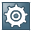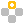Show TOC

###Depreciation AreasIn SAP Business One, you can use different depreciation areas for showing the value of fixed assets for a specific purpose.Example

Balances for tax purposes or management accounting values

End of the example.

SAP Business One supports the following types of depreciation areas:

• Posting to G/L – The area for posting asset depreciations in the general ledger accounts.

• Derived Area – The derived area of the main depreciation area. Generally, you use this area when carrying out special depreciations.

In special depreciation, you can specify the same depreciation type for both the normal and alternative depreciation. This way, the main depreciation area carries out the normal depreciation and the special depreciation while the derived area carries out the normal depreciation only. You can then compare the asset depreciation with and without the special depreciation.

The derived area is used only for information purposes, and there is no posting in the general ledger.

• Additional Area – The area in addition to the posting depreciation areas. You can use this area to compare the effects of using different depreciation areas. You can carry out an additional depreciation calculation for a different depreciation area in parallel to the actual calculation. The parallel calculation is for information purposes only, and no posting is carried out in the general ledger.